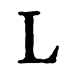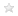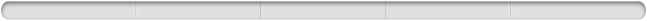Diese Seite verwendet Cookies für unsere Dienste, zur Verbesserung unserer Leistungen, für Analytik und (falls Sie nicht eingeloggt sind) für Werbung. Indem Sie LibraryThing nutzen, erklären Sie dass Sie unsere Nutzungsbedingungen und Datenschutzrichtlinie gelesen und verstanden haben. Die Nutzung unserer Webseite und Dienste unterliegt diesen Richtlinien und Geschäftsbedingungen.
Hide this

Auf ein Miniaturbild klicken, um zu Google Books zu gelangen.

Lädt ...

# Structure of algebras (1939. Auflage)

 Mitglieder Rezensionen Beliebtheit Durchschnittliche Bewertung Diskussionen 13 Keine 1,312,321 Keine Keine The first three chapters of this work contain an exposition of the Wedderburn structure theorems. Chapter IV contains the theory of the commutator subalgebra of a simple subalgebra of a normal simple algebra, the study of automorphisms of a simple algebra, splitting fields, and the index reduction factor theory. The fifth chapter contains the foundation of the theory of crossed products and of their special case, cyclic algebras. The theory of exponents is derived there as well as the consequent factorization of normal division algebras into direct factors of prime-power degree. Chapter VI consists of the study of the abelian group of cyclic systems which is applied in Chapter VII to yield the theory of the structure of direct products of cyclic algebras and the consequent properties of norms in cyclic fields. This chapter is closed with the theory of \$p\$-algebras. In Chapter VIII an exposition is given of the theory of the representations of algebras. The treatment is somewhat novel in that while the recent expositions have used representation theorems to obtain a number of results on algebras, here the theorems on algebras are themselves used in the derivation of results on representations. The presentation has its inspiration in the author's work on the theory of Riemann matrices and is concluded by the introduction to the generalization (by H. Weyl and the author) of that theory. The theory of involutorial simple algebras is derived in Chapter X both for algebras over general fields and over the rational field. The results are also applied in the determination of the structure of the multiplication algebras of all generalized Riemann matrices, a result which is seen in Chapter XI to imply a complete solution of the principal problem on Riemann matrices.… (mehr)
 Mitglied: morphismus Titel: Structure of algebras Autoren: A. Adrian Albert Info: New York, American Mathematical Society, 1939. Sammlungen: Deine Bibliothek Bewertung:Tags: Keine

#### Werk-Informationen

Structure of Algebras von A. Adrian Albert

 Kürzlich hinzugefügt von konyv, morphismus, BYUMath, wilkesmcs, differentia, dominicsoda, hmcmath, appleby, JohnCernes
Keine
Lädt ...Melde dich bei LibraryThing an um herauszufinden, ob du dieses Buch mögen würdest.

Keine aktuelle Diskussion zu diesem Buch.

Keine Rezensionen
Du musst dich einloggen, um "Wissenswertes" zu bearbeiten.
Weitere Hilfe gibt es auf der "Wissenswertes"-Hilfe-Seite.
Gebräuchlichster TitelOriginaltitelAlternative TitelUrsprüngliches ErscheinungsdatumFiguren/CharaktereWichtige SchauplätzeWichtige EreignisseZugehörige FilmePreise und AuszeichnungenEpigraph (Motto/Zitat)WidmungErste WorteZitateLetzte WorteHinweis zur IdentitätsklärungVerlagslektorenWerbezitate vonOriginalspracheAnerkannter DDC/MDSAnerkannter LCCLiteraturhinweise zu diesem Werk aus externen Quellen.

##Wikipedia auf Englisch (3)

 Abraham Adrian AlbertNilpotent algebra Non-associative algebra
The first three chapters of this work contain an exposition of the Wedderburn structure theorems. Chapter IV contains the theory of the commutator subalgebra of a simple subalgebra of a normal simple algebra, the study of automorphisms of a simple algebra, splitting fields, and the index reduction factor theory. The fifth chapter contains the foundation of the theory of crossed products and of their special case, cyclic algebras. The theory of exponents is derived there as well as the consequent factorization of normal division algebras into direct factors of prime-power degree. Chapter VI consists of the study of the abelian group of cyclic systems which is applied in Chapter VII to yield the theory of the structure of direct products of cyclic algebras and the consequent properties of norms in cyclic fields. This chapter is closed with the theory of \$p\$-algebras. In Chapter VIII an exposition is given of the theory of the representations of algebras. The treatment is somewhat novel in that while the recent expositions have used representation theorems to obtain a number of results on algebras, here the theorems on algebras are themselves used in the derivation of results on representations. The presentation has its inspiration in the author's work on the theory of Riemann matrices and is concluded by the introduction to the generalization (by H. Weyl and the author) of that theory. The theory of involutorial simple algebras is derived in Chapter X both for algebras over general fields and over the rational field. The results are also applied in the determination of the structure of the multiplication algebras of all generalized Riemann matrices, a result which is seen in Chapter XI to imply a complete solution of the principal problem on Riemann matrices.

Keine Bibliotheksbeschreibungen gefunden.

BuchbeschreibungZusammenfassung in Haiku-Form## Melvil Decimal System (DDC)

512.24 — Natural sciences and mathematics Mathematics Algebra Groups and groups theory Imaginary expressions

## Bewertung

Durchschnitt: Keine Bewertungen.

## Bist das du?

Werde ein LibraryThing-Autor.

Über uns | Kontakt/Impressum | LibraryThing.com | Datenschutz/Nutzungsbedingungen | Hilfe/FAQs | Blog | LT-Shop | APIs | TinyCat | Nachlassbibliotheken | Vorab-Rezensenten | Wissenswertes | 171,522,443 Bücher! | Menüleiste: Immer sichtbar